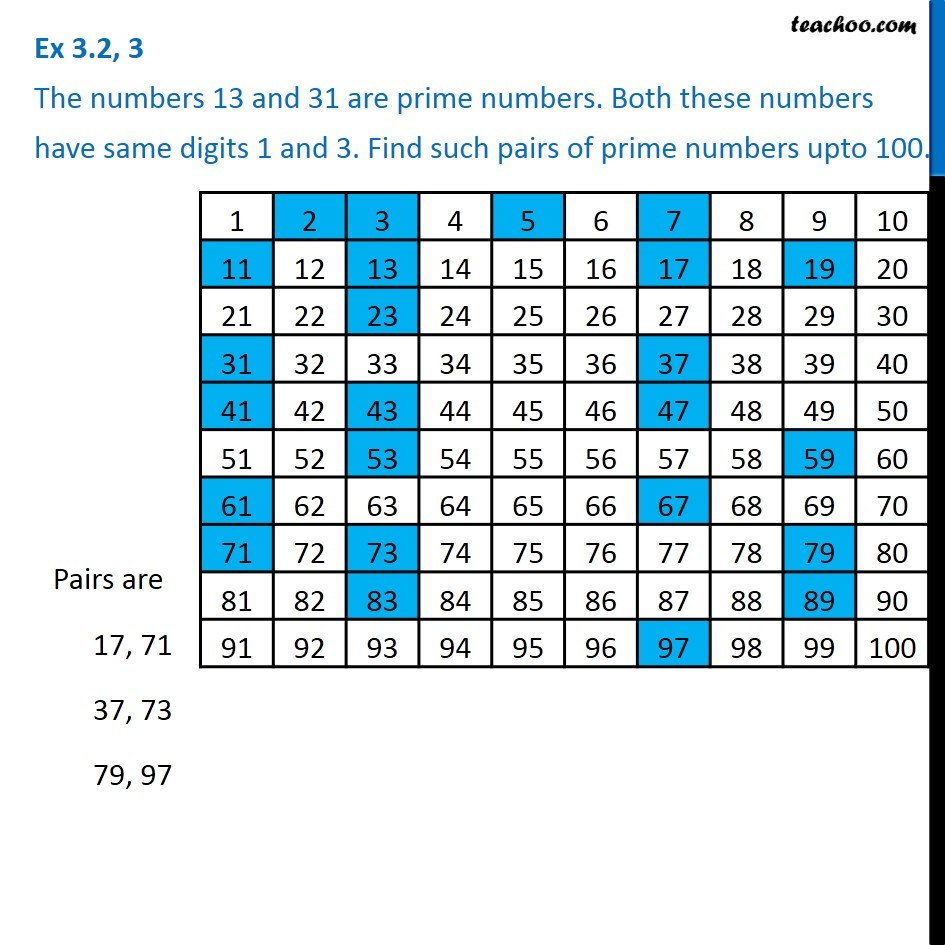1. Chapter 3 Class 6 Playing with Numbers
2. Concept wise
3. Prime and Composite Numbers

Transcript

Ex 3.2, 3 The numbers 13 and 31 are prime numbers. Both these numbers have same digits 1 and 3. Find such pairs of prime numbers upto 100. Prime numbers are numbers which have only 2 factors - 1 and the number itself. Now, lets see a list of numbers from 1 to 100, and mark which are prime numbers. By seeing the list, the numbers which have the same digits, and are prime are 17, 71 37, 73 79, 97

Prime and Composite Numbers